There are many weaknesses of Convonutional Neural Networks (CNN), which Geoffery Hilton mentioned in his famous talk what is wrongs with CNNs?. Recently published paper introduced neural network “CapsuleNet” (also named as “CapsNet”), based on so-called capsules. A capsule is a group of neurons whose output represents different properties of the same entity. These network use Dynamic Routing Between Capsules. CapsuleNet has gained much attention, because it introduces a completely new method, which is most likely to improve overall performance and accuracy of Deep learning algorithms in coming future.

Note: I haven’t covered mathematical equation or programming, but i have mentioned used equation for clarifications.

# CNN: Quick intro

Main component of CNN is convolutional layer, these layers learn and detect main features of an image using pixel input. These layers are stacked up to learn and detect even more complex features. Layers close to input learn simple features whereas higher layer combine simple features into more complex features. Higher-level features combine lower-level features as a weighted sum: activations of a preceding layer are multiplied by the following layer neuron’s weights and added, before being passed to activation nonlinearity. (For more detailed study visit link)

# Difference between CNN and CapsuleNet

• Instead of single neuron, layers of CapsuleNet consist of groups of Neurons called as Capsule. These neurons have same activity vector which represents the instantiation parameters of a specific type of entity such as an object or an object part.
• CNN uses Max pooing for discretization, which is sample-based discretization process. It’s objective is to reduce dimentionality of input representation and reduce chances of overfitting by providing abstract representation of images by allowing neurons in one layer to ignore all but the most active feature detector in a local pool in the layer below [link, paper].
• CapsuleNet uses “Routing by agreement“ approach, in which output is routed to all possible parents but it is scaleddown by coupling coefficients that sum to 1. For each possible parent, the capsule computes a “prediction vector” by multiplying its own output by a weight matrix.
• In standard deep neural networks like AlexNet and ResNet pooling between layers that downsample is a MAX operation. But in CapsuleNet, each layer learns how to pool dynamically and mimic Hebbian learning (more detail in link).
• CapsuleNet is capable of learning to achieve state-of-the art performance by only using a fraction of the data that a CNN would use.

– (1) Pooling in CNN gives away small amount of transitional invariance at each layer, therefore precise location of the most active feature is lost. Due to this reason a CNN classifier can classify Picasso’s “Portrait of woman in d`hermine” as a human face, which isn’t true.
– (2) CNNs cannot extrapolate their understanding of geometric relationships to radically new viewpoints. For example in Diagram 3, there are multiple images of tower of liberty. For a CNN, it is really hard to recognize as same image because it does not have build-in understanding of 3D space, but for a CapsuleNet it is much easier because these relationships are explicitly modeled. The paper which uses this approach was able to cut the error rate by 45% as compared to the previous state of the art, which is a huge improvement [link]. (for more see Geoffery Hilton’s famous his famous talk about what is wrongs with CNNs)Diagram 1: Portrait of woman in d`hermine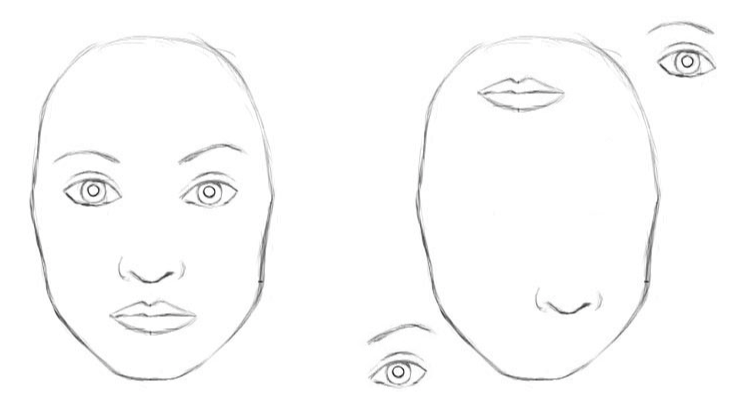Diagram 2: To a CNN, both pictures are similar, since they both contain similar elements.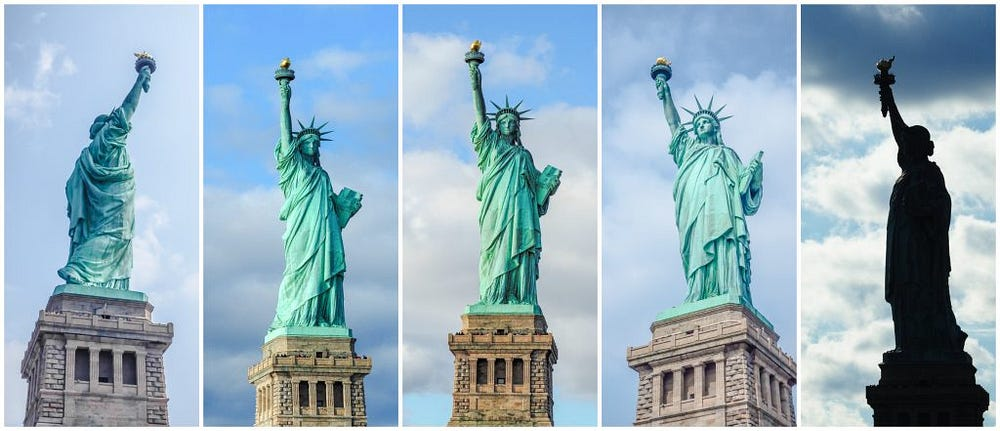Diagram 3: To a CNN, both pictures are similar, since they both contain similar elements.

# CapsuleNet: Network architechture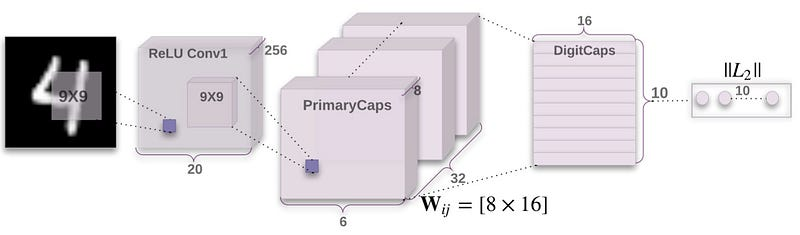Diagram 4: CapsNet, the neural network using capsules

The architecture is shallow with only two convolutional layers and one fully connected layer. Conv1 has 256, 9x9 convolution kernels with a stride of 1 and ReLU activation. This layer converts pixel intensities to the activities of local feature detectors that are then used as inputs to the primary capsules.
The second layer (PrimaryCapsules) is a convolutional capsule layer with 32 channels of convolutional 8D capsules (i.e. each primary capsule contains 8 convolutional units with a 9x9 kernel and a stride of 2). The final Layer (DigitCaps) has one 16D capsule per digit class and each of these capsules receives input from all the capsules in the layer below.

In the above diagram, Dynamic routing occurs between PrimaryCaps and DigitCaps. No routing is used between Conv1 and PrimaryCapsules.

# Calculations

CapsuleNet uses a non-linear “squashing” function to ensure that short vectors get shrunk to almost zero length and long vectors get shrunk to a length slightly below 1 (given in eq 1 in paper). For all but the first layer of capsules, the total input to each capsule is a weighted sum over all “prediction vectors” from the capsules in the layer below which is produced by using eq 2 in paper. The coupling coefficients between a capsule and all the capsules in the layer above sum to 1 and are determined by a “routing softmax”. The initial coupling coefficients are then iteratively refined by measuring the agreement between the current output of each capsule, in the layer above and the prediction made by that capsule. [paper]Diagram 5: pseudo code for the dynamic routing

# Working of a capsule: Simplified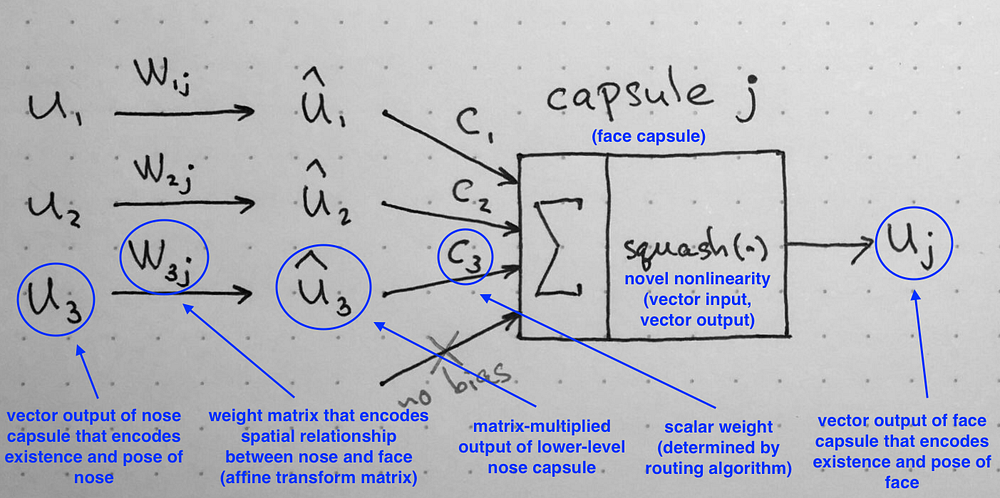Diagram 6: Summary of the internal workings of the capsule. Note that there is no bias because it is already included in the W matrix that can accommodate it and other, more complex transforms and relationships.

The post compares working of traditional neuron and capsule. In order to explain working of capsule let us assume we have a simple model as shown in Diagram 6, which is being used to detect a face. Inputs u1, u2 and u3 are the outputs of capsules from lower layer.

Following are the 4 computational steps happening inside the capsule.

## Matrix multiplication of input vectors

The length of input vectors u1, u2 and u3 corresponds to probability that the lower layer capsules detect objects, and direction of vector corresponds to the internal states of those objects. These vectors are multiplied using corresponding weight matrices W. This weight matrices W, encodes important spatial and other relationships between lower level features and higher level features. In our case lower level features are eyes, nose and mouth, and higher level features is face. After multiplication we get the predicted position of higher level features. In our case vector û1 û2 and û3, represent where face should be according to detected position of eyes, nose and mouth.

## Scalar weighting of input vectors

This step is somewhat similar to the method used in artificial neural networks, where the weights of inputs are learned using backpropagation. In CNN scalar weighting of input vectors is done using max pooling but in Capsules this is done using “Dynamic routing“ (also named as “Routing by agreement“).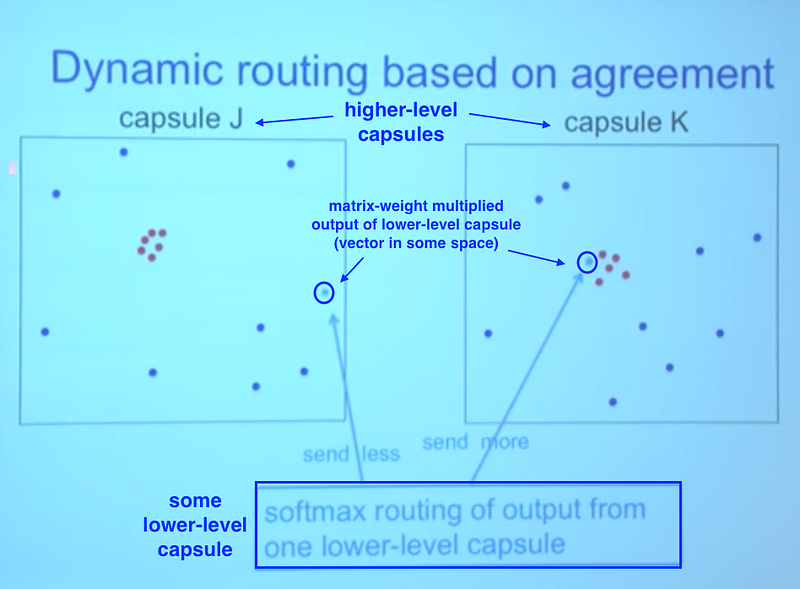Diagram 7: Basics of dynamic routing

In order to understand dynamic routing, lets understand the basic concept first. Initially the lower level capsules sends prior information, then as the time progresses the coupling coefficient gets updated and the capsules with more relevent information forms parse tree. In other words after training process, the capsules with lower features are only connected to those whose information is relevent to representation of higher level capsules. For example images with circle as low level details links with eye or car headlights etc but not probably with fridge. The coupling coefficient for linked capsules becomes slightly less than 1. In Diagram 7, lower level capsule is connected to capsule K, hence the coupling coefficient between lower level capsule and capsule K would be slightly less than 1. (for more indepth explanation read post or watch video)

The paper states:

Initially, the output is routed to all possible parents but is scaled down by coupling coefficients that sum to 1. For each possible parent, the capsule computes a “prediction vector” by multiplying its own output by a weight matrix. If this prediction vector has a large scalar product with the output of a possible parent, there is top-down feedback which increases the coupling coefficient for that parent and decreasing it for other parents.

Top-down feedback is used to update the coupling coefficient of outputs of parent capsules. This coeffient depends on the scalar product of prediction vector and activity vector. In our case, coupling coefficient for capsules 1, 2 and 3 depends on scalar product of û1 and v1, û2 and v2 and û3 and v3. In paper, equation (3) describes the method of calculate coupling coefficient between capsule i and all the capsules in the layer above, where bij is the scalar product of prediction vector of capsule i and activity vector of capsule j from layer l+1. This is refered to as “routing softmax“ in the paper. The value of bij is iteratively refined as shown in Diagram 5 and in link.

## Sum of weighted input vectors

This step is similar to the regular artificial neuron and represents combination of inputs. Sum of weighted input vectors of capsule j, Sj, can be calculated using eqation (2) of paper, which is summation of product of coupling coefficient and input vectors.

## Vector-to-vector nonlinearity

This is another unique approach introduced in CapsuleNet, it uses non-linear activation function, refered to as squash function in Diagram 5. This function ensures that short vectors get shrunk to almost zero length and long vectors get shrunk to a length slightly below 1.

Resources:
I have used a lot of images and concepts from this post, paper and video. For code visit link1 and link2.